Next: Known SSC Results for Up: Sufficient Optimality for Discretized Previous: Introduction

# Parabolic and Elliptic Control Problems

In this section we describe the continuous control problems exemplarily considered in the following. The first is a one-dimensional parabolic boundary control problem formulated such that it includes problems from [1,9] and has additional pointwise state constraints.

minimize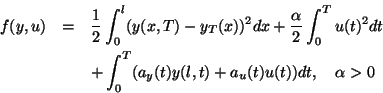subject to(P)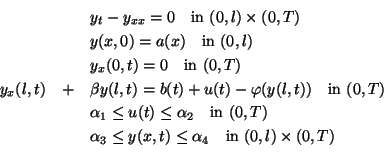The notation above is that of  and the corresponding data will be defined in section 5 below. The problem does, however, include also one considered in , namely by choosing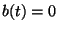,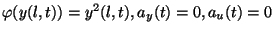.

We define the following discretization of problem (P).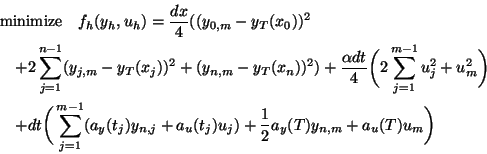subject to(P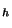)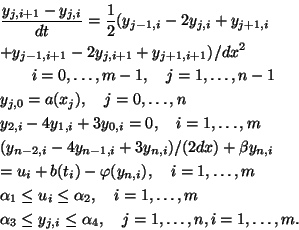Here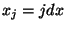,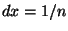,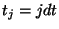,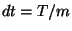.

For the problem (P) above and specific data an analytic solution is given in  and this also permits the authors to verify the necessary and sufficient optimality conditions they had stated and proved.

Next, we consider the instationary Burgers equation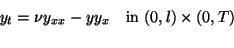which we discretize by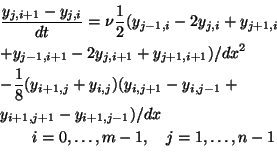For the elliptic control problems we consider the class defined in . It includes boundary and distributed controls which are addressed separately in , respectively  as well as Dirichlet, Neumann, and mixed boundary conditions. In the case of boundary control the underlying continuous problem is

minimize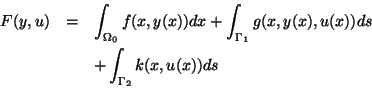subject to(EB)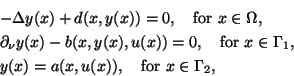and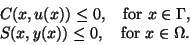Here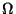is a bounded, plane domain with piecewise smooth boundary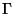.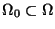is equal tounless noted otherwise.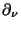denotes the derivative in the direction of the outward unit normal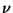onand the boundary is partitioned as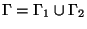with disjoint sets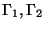consisting of finitely many connected components. For the general formulation given above in [17,18] necessary optimality conditions are stated, a discretization is described in full detail and the corresponding optimality conditions are related carefully to those for the continuous problem. With concrete applications in mind then, however, the following data of the problem are specialized, the objective function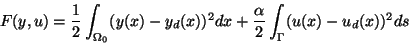(2.1)

with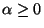and the inequality constraints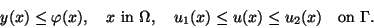(2.2)Next: Known SSC Results for Up: Sufficient Optimality for Discretized Previous: Introduction
Hans Mittelmann
2000-08-31